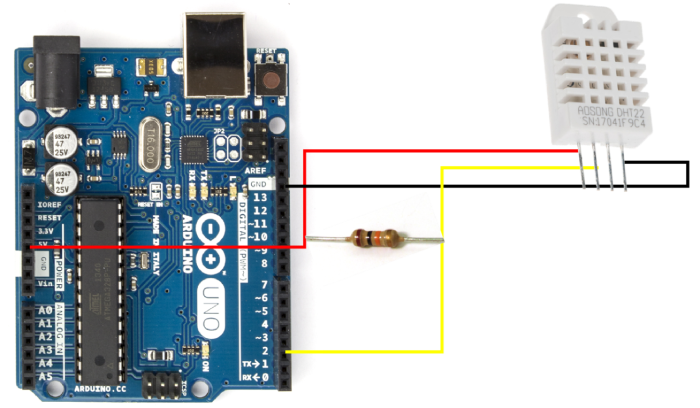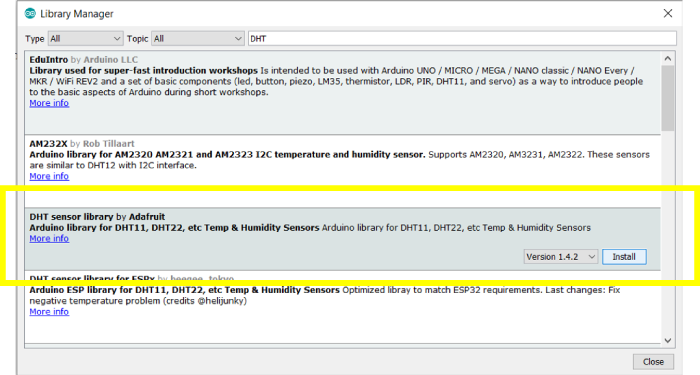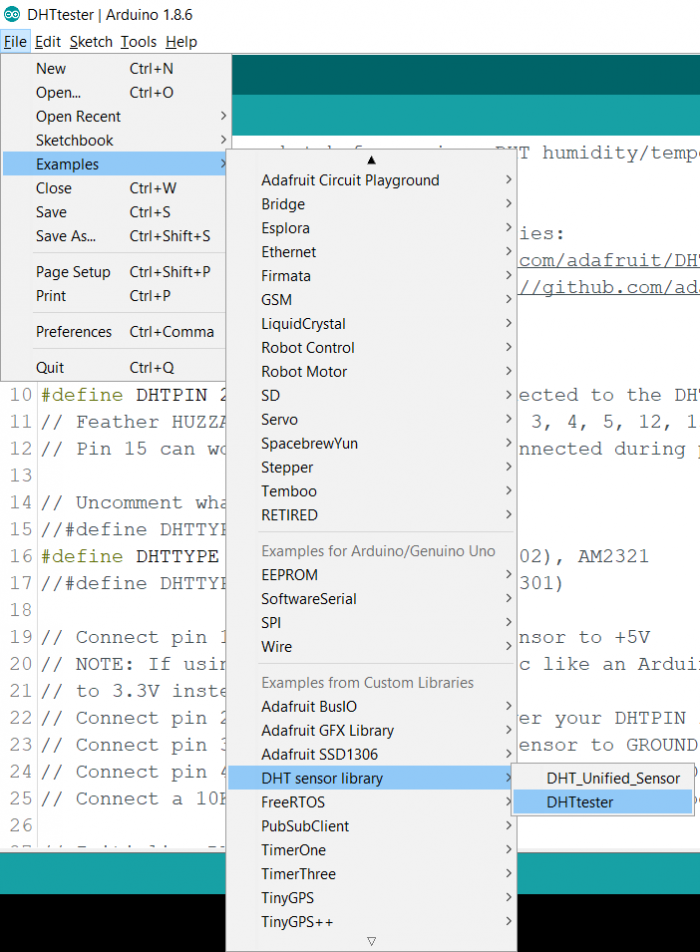# Getting data from temperature and humidity sensor using Arduino

ArduinoArduino BoardsArduino IDEArduino Programming Language

In this tutorial, we will interface Arduino DHT-22 Temperature and Humidity Sensor and print the obtained temperature and humidity values on the Serial Monitor.

## Circuit DiagramWhen the DHT-22 is facing you, the first pin from the left, the VCC pin is connected to 5V, the next pin is the DATA pin, and it is connected to pin 2 on Arduino Uno. The third pin is Not Connected. The 4th pin, GND, is connected to GND on Arduino. A 10K resistor is to be connected between the DATA pin and the Vcc pin of DHT22, as you can see in the above diagram.

## Required Libraries

The DHT Sensor Library by Adafruit library will be required for interfacing Arduino Uno with the OLED Display −

Go to Tools → Manage Libraries, search for this library, and click Install.## Code Walkthrough

We will walkthrough an example code that comes along with the DHT sensor library. Go to File → Examples → DHT sensor library → DHTtesterAlternatively, the code can be accessed on GitHub here − https://github.com/adafruit/DHTsensor-library/blob/master/examples/DHTtester/DHTtester.ino

As you can see, we begin with the inclusion of the DHT library.

#include "DHT.h"

Next, we define the digital pin that is connected to the DHT sensor (pin 2 of Arduino in this case). And we also define the type of DHT sensor that we are using (DHT22)

#define DHTPIN 2
#define DHTTYPE DHT22

Next, we define the DHT object, using the DHTPIN and DHTTYPE defined earlier

DHT dht(DHTPIN, DHTTYPE);

Within the setup, we initialize Serial, and also the dht, using dht.begin().

void setup() {
Serial.begin(9600);
Serial.println(F("DHTxx test!"));

dht.begin();
}

Within the loop, we first add a delay of 2 seconds. That enables some time interval between two readings. Next, we read the humidity and temperature using the .readHumidity() and .readTemperature() functions. The .readTemperature() function takes in a Boolean argument, which, when set to true, returns the temperature in Fahrenheit (the return is in Celsius by default).

If any of the 3 readings are NaN, we return (you can also write continue here). Finally, we compute the heat index using the read temperature and humidity values. You can read more about the heat index here .

Finally, we print all the read/ calculated values.

void loop() {
// Wait a few seconds between measurements.
delay(2000);

// Sensor readings may also be up to 2 seconds 'old' (its a very slow sensor)
// Read temperature as Celsius (the default)
// Read temperature as Fahrenheit (isFahrenheit = true)

// Check if any reads failed and exit early (to try again).
if (isnan(h) || isnan(t) || isnan(f)) {
Serial.println(F("Failed to read from DHT sensor!"));
return;
}

// Compute heat index in Fahrenheit (the default)
float hif = dht.computeHeatIndex(f, h);
// Compute heat index in Celsius (isFahreheit = false)
float hic = dht.computeHeatIndex(t, h, false);

Serial.print(F("Humidity: "));
Serial.print(h);
Serial.print(F("% Temperature: "));
Serial.print(t);
Serial.print(F("°C "));
Serial.print(f);
Serial.print(F("°F Heat index: "));
Serial.print(hic);
Serial.print(F("°C "));
Serial.print(hif);
Serial.println(F("°F"));
}

Upload this code to your Arduino and you will be able to observe the temperature and humidity values on the Serial Monitor.